Next: 6.3 Amigos Up: 6. Further Applications of Previous: 6.1 MINIMOS-NT

# 6.2 Levenberg-Marquardt Optimizer

The Algorithm Library is used to equip the Levenberg-Marquardt Optimizer mlmmin  with an interface to the MDL language to define analytical functions whose parameters will be fitted to match experimental data sets contained in results from TCAD simulations. For that purpose a TCAD engineer has to provide an IPL input deck which defines optimization parameter names, their starting values, and optionally their allowed numerical ranges. Furthermore the names of variables which are associated with complying data columns and the file names of an MDL file and the data set have to be defined. The therein provided parameters are forwarded to the Interface of mlmmin specific Model class FunctionModel within the associative map ue. The function whose parameters are fitted to match with the data set has to be defined within the evaluate method of the fit_function Model instance which has to be derived from FunctionModel.

Example 6.3 and 6.4 demonstrate the application of this tool for automatically extracting the parameters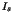(Is) and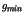(Gmin) of the discrete large signal model of a diode (6.1) from a parameter set diode.dat containing pairs of(Id) and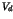(Vd) values obtained by device simulations with MINIMOS-NT. The parameters section of the IPL input deck (Example 6.3) introduces the desired parameters and provides their start values for the fitting process. The data structure of the diode.ipd file is described in the dataset section to contain a column forvalues and a second one for the results of the function to be fitted to these data values.

The fit function (6.1) defined within diode.mdl (Example 6.4) uses local Parameters (Is, Vd, and Gmin) to simplify the notation of the evaluate method which computes its result for a assumed temperature of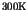.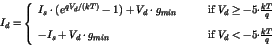(6.1)parameters {  // define optimization parameters
Is   { nom=1e-14.;}
Gmin { nom=1e20; }
}

dataset { // values conatained in the data set
filename="diode.dat";
variables { Vd {}; } // {} defaults to "result"
}

model {
file="diode.mdl";
}

Example 6.3: IPL file for the Levenberg-Marquardt optimizerInstance fit_function = DiodeFitModel;
NewModel DiodeFitModel : FunctionModel {
Local {
// assume T=300 [K]
Parameter<double> qdkT = 1.60218e-19 / 1.38066e-23 / 300.0;
Parameter<double> Is;
Parameter<double> Gmin;
Parameter<double> Vd;
}

evaluate {
Is   = :Ue["Is"];
Vd   = :ue["Vd"];
Gmin = :ue["Gmin"];

if (Vd >= (-5*qdkT)) {
:result = Is * exp ( Vd*qdkt ) + Vd*Gmin;
} else {
:result = -Is + Vd*Gmin;
}
}
}

Example 6.4: MDL file for the Levenberg-Marquardt optimizer

It should also be mentioned that the implementation of the mlmmin optimizer serves as an example for the usage of the multi language binding mechanisms of the Algorithm Library in conjunction with the VMAKE Tool Abstraction Concept (TAC). mlmmin consists of a kernel written in C, optimization algorithms contained in a FORTRAN library, an user interface implemented by utilizing the Vienna LISP'' interpreter and the Algorithm Library extension implemented in C++ which exchange data via automatically generated TAC language bindings.Next: 6.3 Amigos Up: 6. Further Applications of Previous: 6.1 MINIMOS-NT
Robert Mlekus
1999-11-14